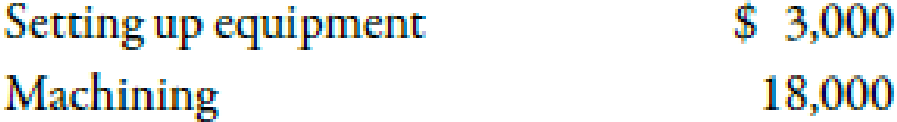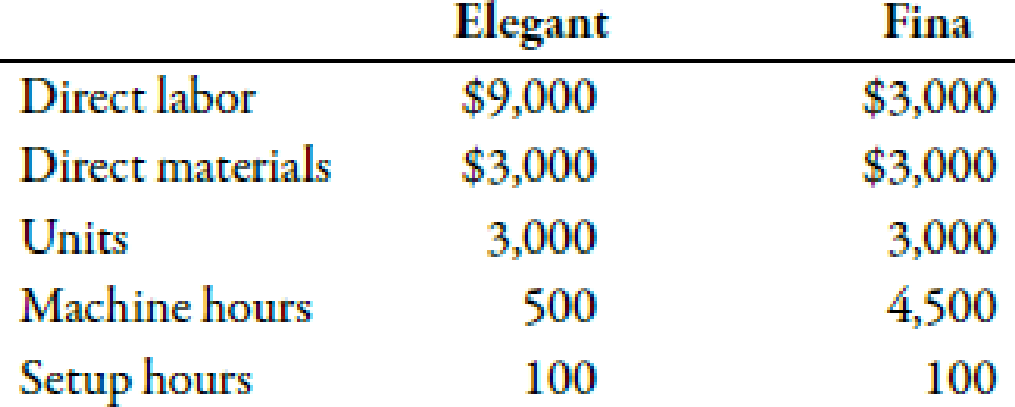Chapter 5, Problem 48E### Managerial Accounting: The Corners...

7th Edition
Maryanne M. Mowen + 2 others
ISBN: 9781337115773

#### Solutions

Chapter
Section### Managerial Accounting: The Corners...

7th Edition
Maryanne M. Mowen + 2 others
ISBN: 9781337115773
Textbook Problem
134 views

# Product-Costing Accuracy, Consumption RatiosPlata Company produces two products: a mostly handcrafted soft leather briefcase sold under the label Maletin Elegant and a leather briefcase produced largely through automation and sold under the label Maletin Fina. The two products use two overhead activities, with the following costs:The controller has collected the expected annual prime costs for each briefcase, the machine hours, the setup hours, and the expected production.Required: 1. CONCEPTUAL CONNECTION Do you think that the direct labor costs and direct materials costs are accurately traced to each briefcase? Explain. 2. Calculate the consumption ratios for each activity. Round to two decimal places. 3. Calculate the overhead cost per unit for each briefcase by using a plantwide rate based on direct labor costs. Round rates to the nearest cent. Comment on this approach to assigning overhead. 4. CONCEPTUAL CONNECTION Calculate the overhead cost per unit for each briefcase by using overhead rates based on machine hours and setup hours. Explain why these assignments are more accurate than those using the direct labor costs.

1.

To determine

Identify whether the direct labor costs and direct material costs are accurately traced to each briefcase. Also, explain the reason.

Explanation

Consumption Ratio:

Consumption ratio is the fraction of each activity that is consumed by a product. Activity drivers are used to calculate the activity output, and then it is further used to calculate the activity consumption...

2.

To determine

Compute the consumption ratios for each activity.

3.

To determine

Compute the overhead cost per unit for each briefcase with the help of plant wide overhead rate based on direct labor costs. Also, explain the approach to assigning overhead.

4.

To determine

Compute the overhead cost per unit for each briefcase by using overhead rates based on machine hours and setup hours.

### Still sussing out bartleby?

Check out a sample textbook solution.

See a sample solution

#### The Solution to Your Study Problems

Bartleby provides explanations to thousands of textbook problems written by our experts, many with advanced degrees!

Get Started

#### Find more solutions based on key concepts